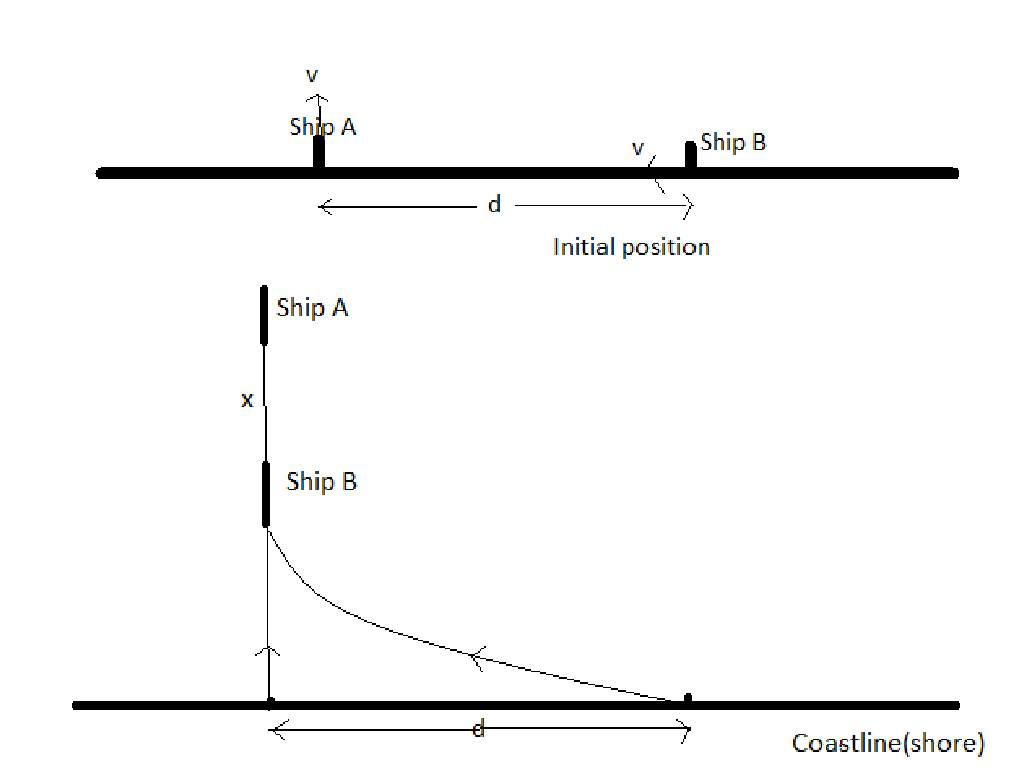# Catch the Thief!Two ships $A$ and $B$ are originally at a distance $d$ from each other depart at the same time from a straight coastline. Ship $A$ moves along a straight line perpendicular to the shore while ship $B$ constantly heads for ship $A$, having at the each moment same as the latter. After a sufficiently great interval of time the second ship will be following the first one at a certain distance $x$. Find $x$ in the image above.

Details and Assumptions

• $d= 26$ m

• In final position they are in same straight line perpendicular to coastline ( track of $A$ )

• Their speeds are the same.

×

Problem Loading...

Note Loading...

Set Loading...